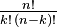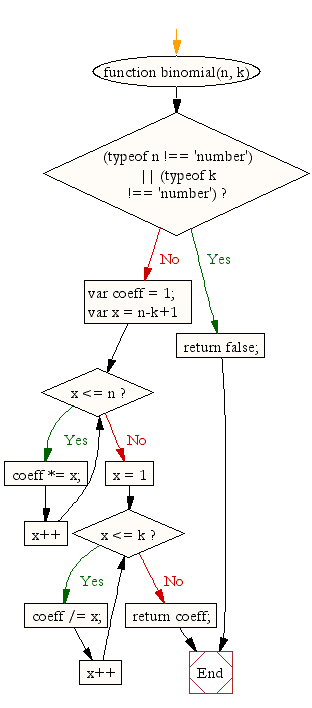# JavaScript: Evaluate binomial coefficients

## JavaScript Math: Exercise-20 with Solution

Write a JavaScript program to evaluate binomial coefficients.

Note:
Binomial coefficient : According to Wikipedia - In mathematics, binomial coefficients are a family of positive integers that occur as coefficients in the binomial theorem. They are indexed by two nonnegative integers; the binomial coefficient indexed by n and k is usually written \tbinom nk. It is the coefficient of the x k term in the polynomial expansion of the binomial power (1 + x) n. Under suitable circumstances the value of the coefficient is given by the expression :Arranging binomial coefficients into rows for successive values of n, and in which k ranges from 0 to n, gives a triangular array called Pascal's triangle.

Test Data:
console.log(binomial(8,3));
console.log(binomial(10,2));
Output :
56
45

Sample Solution:-

HTML Code:

``````<!DOCTYPE html>
<html>
<meta charset="utf-8">
<title>Binomial coefficient</title>
<body>
</body>
</html>
```
```

JavaScript Code:

``````function binomial(n, k) {
if ((typeof n !== 'number') || (typeof k !== 'number'))
return false;
var coeff = 1;
for (var x = n-k+1; x <= n; x++) coeff *= x;
for (x = 1; x <= k; x++) coeff /= x;
return coeff;
}

console.log(binomial(8,3));
console.log(binomial(10,2));
```
```

Sample Output:

```56
45
```

Flowchar:Live Demo:

See the Pen javascript-math-exercise-20 by w3resource (@w3resource) on CodePen.

Improve this sample solution and post your code through Disqus

What is the difficulty level of this exercise?

Test your Programming skills with w3resource's quiz.

﻿